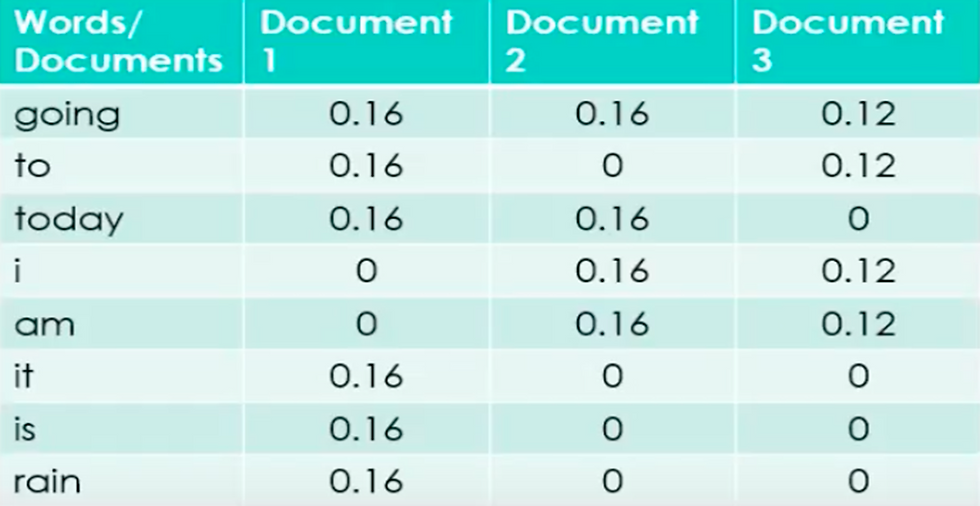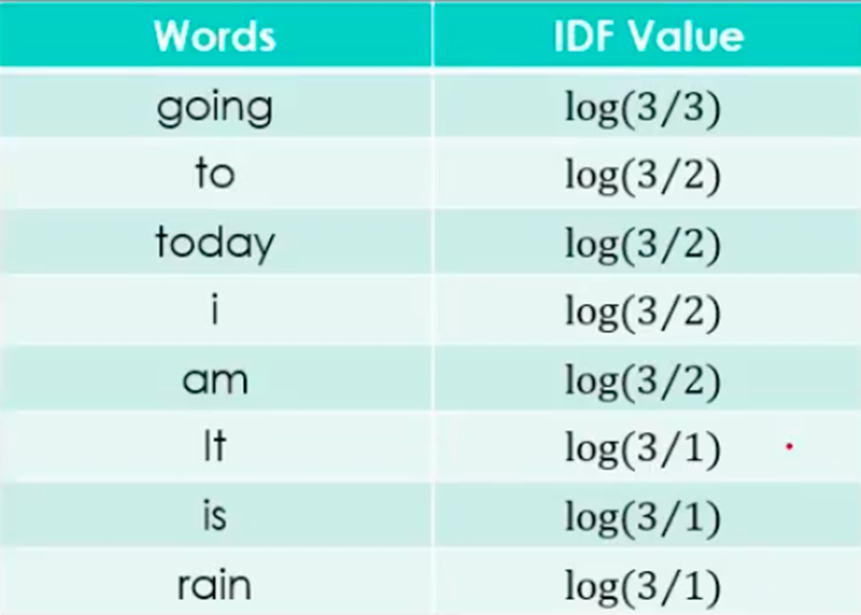top of page
Search

# Term Frequency(TF) and Inverse Document Frequency (IDF) | What is TF and IDF? | Realcode4you

What is Term Frequency(TF)?

- Assign each term in a document a weight for that term.

- The weight of a term t in a document d is a function of the number of times t appears in d.

• The weight can be simply set to the number of occurrences of t in d :

tf (t, d) = count (t, d)

• The term frequency may optionally be normalized.

What is Inverse Document Frequency (idf)

idf(t) = log [N/df(t)]

• N: Number of documents in the corpus

• df(t): Number of documents in the corpus that contain a term t

- Measures term uniqueness in corpus

• "phone" vs. "brick"

- Indicates the importance of the term

• Search (relevance)

• Classification (discriminatory power)

TF-IDF and Modified Retrieval Algorithm

- term t in document d:

tfidf(t, d) = tf (t, d) * idf(t)

query: brick, phone

• Document with "brick" a few times more relevant than document with "phone" many times

• Measure of Relevance with tf-idf

• Call up all the documents that have any of the terms from the query, and sum up the tf-idf of each term:TF-IDF and Modified Retrieval Algorithm, example

• The process to find meaning of documents using TF-IDF is very similar to Bag of words,

• Clean data / Preprocessing — Clean data (standardise data) , Normalize data( all lower case) , lemmatize data ( all words to root words ).

• Tokenize words with frequency

• Find TF for words

• Find IDF for words

• Vectorize vocab

Example

• Let’s cover an example of 3 documents -

• Document 1 It is going to rain today. 1/6

• Document 2 Today I am not going outside.

• Document 3 I am going to watch the season premiere. 1/8

To find TF-IDF we need to perform the steps we laid out above, let’s get to it.

• Step 1 Clean data and TokenizeExample, continue

- Step 2: Find TF for all docs

`TF = (Number of repetitions of word in a document) / (# of words in a document)`- Step 3: Find IDF

`IDF =Log[(Number of documents) / (Number of documents containing the word)]`

In Excel use LN(3/3)- Step 4: Build model i.e. stack all words next to each otherIDF Value and TF value of 3 documents.

- Step 5: Compare results and use table to ask questionsRemember, the final equation = TF-IDF = TF * IDF

Example, continue- Analysis and outcomes

• You can easily see using this table that words like ‘it’,’is’,’rain’ are important for document 1 but not for document 2 and document 3 which means Document 1 and 2&3 are different w.r.t talking about rain.

• You can also say that Document 1 and 2 talk about something ‘today’, and document 2 and 3 discuss something about the writer because of the word ‘I’.

• This table helps you find similarities and non similarities between documents, words and more much better than Bag Of Words.